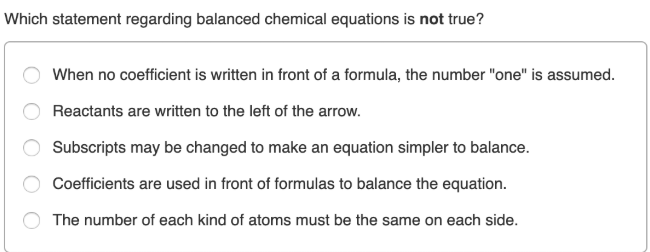# Which statement regarding balanced chemical equations is not true? a. When no coefficient is written in front of a formula, the number "one" is assumed. b. Reactants are written to the left of the arrow. c. Subscripts may be changed to make an equation simpler to balance. d. Coefficients are used in front of formulas to balance the equation. e. The number of each kind of atoms must be the same on each side.